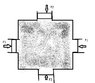# Pascal law of fluids

• jonny23

## Homework Statement

a water filled symetrical container has four pistons , one on each side of area A to keep water in equilibrium

Now an additional force F is applied to all four pistons . then increase in pressure at the middle of container will be:

## Homework Equations

pascal law: change is pressure is transmitted to whole fluid

## The Attempt at a Solution

since F is applied from four sides so pressure should have been 4F/A but the solution says F/A

#### Attachments

•Untitled.jpg
69 KB · Views: 391

What's the definition of pressure?

Perpendicular force per unit area..

Now apply that definition to the problem.

but the force is from all sides so either vector sum should be 0
or if add as scalar it should be 4F/A...
but the answer is only F/A

The force is being applied to a fluid, not a solid. What happens when you stand on a water balloon?

can you give a more detailed reply to this question...

Perpendicular force per unit area..
but the answer is only F/A
4F/4A is the same thing as F/A. If we're discussing pressure as a thermodynamic variable, it's what is called an intensive variable, independent of the size of a system. Mathematically, it's a scalar. Force has a direction, yes; force per unit area has no direction. Pressure times area has a direction. The scalar-vector conflict may be what's confusing you. Stick with it, and we'll sort it out for you.

•jonny23